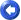﻿ Technical Indicators# Technical Indicators

• Simple Moving Average

The Simple Moving Average is simply an average of values over a specified period of time.

Interpretation

A Moving Average is most often used to average values for a smoother representation of the underlying price or indicator.

• Exponential Moving Average

An Exponential Moving Average is similar to a Simple Moving Average. An EMA is calculated by applying a small percentage of the current value to the previous value. An EMA applies more weight to recent values.

Interpretation

A Moving Average is most often used to average values for a smoother representation of the underlying price or indicator.

• Weighted Moving Average

A Weighted Moving Average places more weight on recent values and less weight on older values.

### Interpretation

A Moving Average is most often used to average values for a smoother representation of the underlying price or indicator.

• Williams’ %R

Williams’ %R measures overbought/oversold levels.

### Interpretation

The most widely used method for interpreting Williams’ %R is to buy when the indicator rises above 80 or sell when the indicator falls below 20.

• True Range

The Average True Range (Wilder) measures market volatility.

Interpretation

High ATR values may signal market bottoms, and low ATR values may signal neutral markets.

• Parabolic SAR

The Parabolic SAR was  developed by Welles Wilder. This indicator is always in the market (whenever a position is closed, an opposing position is taken).  The Parabolic SAR indicator is most often used to set trailing price stops. A stop and reversal (SAR) occurs when the price penetrates a  Parabolic SAR level.

• Momentum Oscillator

Momentum Oscillator (Chande) is an advanced momentum oscillator derived from linear regression.

Interpretation

Increasingly high values of CMO may indicate that prices are trending strongly upwards. Conversely, increasingly low values of CMO may indicate that prices are trending strongly downwards. CMO is related to MACD and Price Rate of Change (ROC).

• Moving Average Convergence / Divergence (MACD)

The MACD is a moving average oscillator that shows potential overbought/oversold phases of market fluctuation. The MACD is a calculation of two moving averages of the underlying price/indicator.

### Interpretation

Buy/Sell interpretations may be derived from crossovers (calculated from the Signal Periods parameter), overbought/oversold levels of the MACD and divergences between MACD and actual price.

• Price Oscillator

The Price Oscillator shows a spread of two moving averages.

### Interpretation

The Price Oscillator is basically a moving average spread. Buying usually occurs when the oscillator rises, and conversely, selling usually occurs when the oscillator falls.

• Standard Deviation

Standard Deviation is a common statistical calculation that measures volatility. Other technical indicators are often calculated using standard deviations.

Interpretation

Major highs and lows often accompany extreme volatility. High values of standard deviations indicate that the price or indicator is more volatile than usual.

• Price Rate of Change

The Price ROC shows the difference between the current price and the price one or more periods in the past.

### Interpretation

A 12-day Price ROC is most often used as an overbought/oversold indicator.

• Stochastic Oscillator

The Stochastic Oscillator is a popular indicator that shows where a security’s price has closed in proportion to its closing price range over a specified period of time.

### Interpretation

The Stochastic Oscillator has two components: %K and %D. %K is most often displayed as a solid line and %D is often shown as a dotted line. The most widely used method for interpreting the Stochastic Oscillator is to buy when either component rises above 80 or sell when either component falls below 20. Another way to interpret the Stochastic Oscillator is to buy when %K rises above %D, and conversely, sell when %K falls below %D.

The most commonly used arguments are 9 for %K periods, 3 for %K slowing periods and 3 for %D smoothing.

• Volume Oscillator

The Volume Oscillator shows a spread of two different moving averages of volume over a specified period of time.

### Interpretation

The Volume Oscillator offers a clear view of whether or not volume is increasing or decreasing.

• Weighted Close

Weighted Close is an average of each day’s open, high, low, and close, where more weight is placed on the close.

### Interpretation

The Weighted Close indicator is a simple method that offers a simplistic view of market prices.